• //DFS深度优先遍历 打印数组的排列情况 4不能在第三位，3，5不能相连 package com.qyc.stringArithmetic; import java.util.Arrays; import java.util.HashSet; import java.util.Iterator; import ...
按要求打印数组的排列情况

要求：“4”不能在第三位，“3”，“5”不能相连

	//DFS深度优先遍历 打印数组的排列情况 4不能在第三位，3，5不能相连
package com.qyc.stringArithmetic;

import java.util.Arrays;
import java.util.HashSet;
import java.util.Iterator;
import java.util.Set;

import org.hamcrest.core.CombinableMatcher.CombinableBothMatcher;
import org.junit.Test;

public class String_Arithmetic {
private int [] numbers= new int []{1,2,2,3,4,5};
private int n = numbers.length;

private boolean visited[] = new boolean[n];
private int[][] graph = new int [n][n];
private String combination = "";

public void buildGraph() {

for(int i=0;i<n;i++){
for(int j = 0;j<n;j++){

if(i == j){
graph[i][j] = 0;
}else{
graph[i][j] = 1;
}
}
}
graph = 0;
graph = 0;
}

public Set<String> getAllCombination() {
buildGraph();
Set<String> set = new HashSet<String>();
for(int i=0;i<n;i++){
this.deptFirstSearch(i, set);
}
return set;

}

public void deptFirstSearch(int start,Set<String> set) {
visited[start] = true;
combination = combination+numbers[start];
if(combination.length()==n){
if(combination.indexOf("4")!=2){
set.add(combination);
}
}
for(int j=0;j<n;j++){
if(graph[start][j]==1&&visited[j]==false){
deptFirstSearch(j, set);
}
}
combination=combination.substring(0,combination.length()-1);
visited[start] = false;
}
public static void main(String[] args) {
String_Arithmetic string_Arithmetic = new String_Arithmetic();
Set<String> set = string_Arithmetic.getAllCombination();
Iterator<String> iterator = set.iterator();
while (iterator.hasNext()) {
String aString = (String)iterator.next();
System.out.println(aString);
}

}
}


展开全文• 图的样子： DFS.c #include ...图的深度优先遍历 vertex是邻接矩阵，每一行代表一个顶点，每一个元素代表与其它顶点是否有连接。 color是一个染色数组 用于区分 是否已经走过这个vertex. DF
图的样子：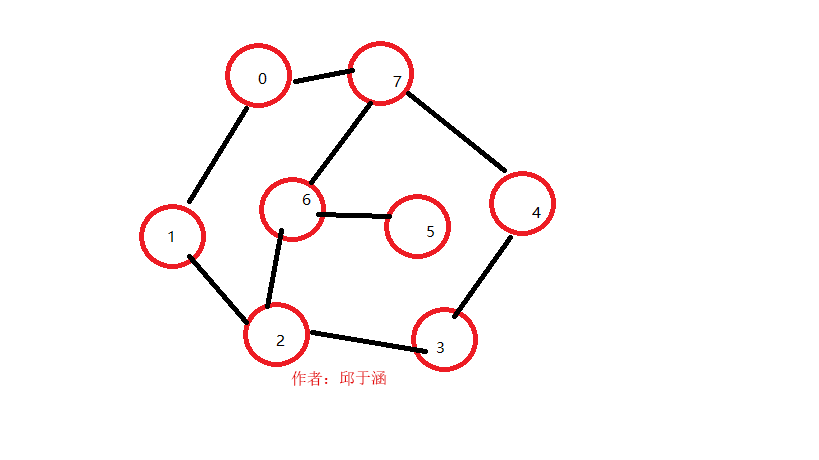DFS.c
#include <stdio.h>
/**************************************
CopyRight(c) 2016-8-30 11:02:57
Author:邱于涵
图的深度优先遍历
vertex是邻接矩阵，每一行代表一个顶点，每一个元素代表与其它顶点是否有连接。
color是一个染色数组 用于区分 是否已经走过这个vertex.
DFS最后一个参数是传入顶点（第几个）
每次需要遍历 完所有 这行的所有顶点（是否为1而且color[x]=0）就递归

***********************************/
//邻接矩阵
int vertex = {
{ 0, 1, 0, 0, 0, 0, 0, 1 },
{ 1, 0, 1, 0, 0, 0, 0, 0 },
{ 0, 1, 0, 1, 0, 0, 1, 0 },
{ 0, 0, 1, 0, 1, 0, 0, 0 },
{ 0, 0, 0, 1, 0, 0, 0, 1 },
{ 0, 0, 0, 0, 0, 0, 1, 0 },
{ 0, 0, 1, 0, 0, 1, 0, 1 },
{ 1, 0, 0, 0, 1, 0, 1, 0 },
};
//染色数组
int color = { 0, 0, 0, 0, 0, 0, 0, 0 };
//深度优先遍历 递归函数
void DFS(int  arr,int * color,int begin);
int main(void)
{
//从第0个开始
DFS(vertex, color, 0);
printf("遍历结束\n");
while (1);
}
//实现
void DFS(int arr, int * color, int i){

//输出当前是第几个顶点
printf("当前正在访问 结点：%d \n", i);
//更改眼色
*(color + i) = 1;

//把这个顶点  的所有  连接点 都遍历（条件是 没有染色 有连接点，注意 j=0是为了全部遍历）
for (int j = 0; j < 8; j++){
if (arr[i][j]==1 && color[j]==0)
//递归
DFS(vertex, color, j);

}
}
如果从0开始遍历，下面是遍历结果：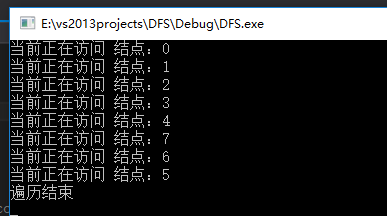如果从 5遍历 ，下面是遍历结果：原创文章，未经允许请勿转载在，本人QQ：1031893464，邱于涵

展开全文数据结构
• DFS深度优先遍历算法，VS2010环境，可运行，数据是自己随便编的
• poj 2488——dfs深度优先遍历 //给行数列数，求问能否遍历，给出字典序的一种遍历
• 数据结构DFS深度优先遍历非递归算法实现，是自己编写的，可靠。
• DFS深度优先遍历算法详解与实现 概括：深度优先是沿着一条路一直走到底，然后进行回溯 该算法是基于图的邻接表存储实现的 图的邻接表存储方式 注意，邻接表中边表节点中存储的值是该节点在数组中的索引值，而...
DFS深度优先遍历算法详解与实现

概括：深度优先是沿着一条路一直走到底，然后进行回溯

该算法是基于图的邻接表存储实现的

图的邻接表存储方式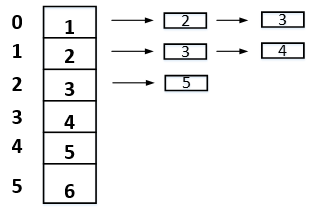注意，邻接表中边表节点中存储的值是该节点在数组中的索引值，而顶点表节点存储的是数据。

更多关于邻接表内容：

https://blog.csdn.net/ASCIIdragon/article/details/84635236

使用一个一维数组visited标记访问过的顶点，对应的下标的元素为1（代表已经被访问），0（代表没有被访问）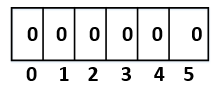step1：

v 是第一个需要访问的顶点在顶点表中的索引值，注意，是索引值。假设v = 0，则将visited标为1.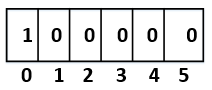step2：

p是指向顶点表[v]的邻接点指针。p = vertices[v].firstarc.

注意，p不是从p = vertices[v]开始的，而是从某顶点的邻接点。p = vertices[v].firstarc.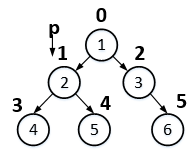step3：

判断p是否为空

不为空，则v  = p->adjvex节点可以访问，递归到step1，将visited = 1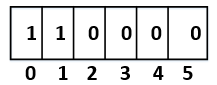然后继续访问v的邻接点，p = vertices[v].firstarc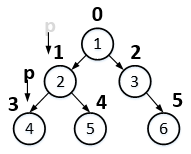如果p不为空，则v  = p->adjvex节点也访问过了，visited = 1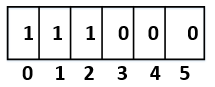然后继续访问v的邻接点，p = vertices[v].firstarc

此时vertices.first为空，则p指向上一次访问的顶点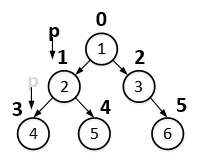然后执行step3，step1，step2，step3......

程序不断重复执行step1，step2，step3，可以用递归的方式实现。

c语言实现代码

//访问标志数组
int visited[MAX] = {0};

//用邻接表方式实现深度优先搜索(递归方式）
//v 传入的是第一个需要访问的顶点索引值
void DFS(MGraph G, int v)
{
//图的顶点的搜索指针
ArcNode *p;
//置已访问标记
visited[v] = 1;
//输出被访问顶点的编号
printf("%d  ", v);
//p指向顶点v的第一条弧的弧头结点
p = G.vertices[v].firstarc;
while (p != NULL)
{
//若p->adjvex顶点未访问,递归访问它
if (visited[p->adjvex] == 0)
{
DFS(G, p->adjvex);
}
//p指向顶点v的下一条弧的弧头结点
p = p->nextarc;
}
}

参考博客：

https://www.cnblogs.com/kubixuesheng/p/4399705.html

https://www.cnblogs.com/kubixuesheng/p/4399705.html（堆栈）
展开全文• DFS 深度优先遍历 DFS算法用于遍历图结构，旨在遍历每一个结点，顾名思义，这种方法把遍历的重点放在深度上，什么意思呢？就是在访问过的结点做标记的前提下，一条路走到天黑，我们都知道当每一个结点都有很多分支，...
DFS 深度优先遍历
DFS算法用于遍历图结构，旨在遍历每一个结点，顾名思义，这种方法把遍历的重点放在深度上，什么意思呢？就是在访问过的结点做标记的前提下，一条路走到天黑，我们都知道当每一个结点都有很多分支，那么我们的小人就沿着每一个结点走，定一个标准，比如优先走右手边的路，然后在到达下一个结点前先敲敲门，当一个结点的所有门都被敲了个遍都标记过，那么就走回头路，再重复敲门，直到返回起点，这样的方式我们叫做 DFS 深度优先遍历，本文以图结构讲解，例子取自《大话数据结构》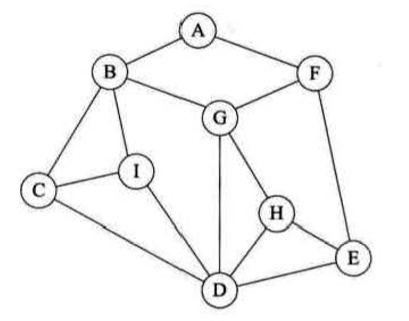如我刚才所讲，从A点出发，将路径画出来就是以下效果。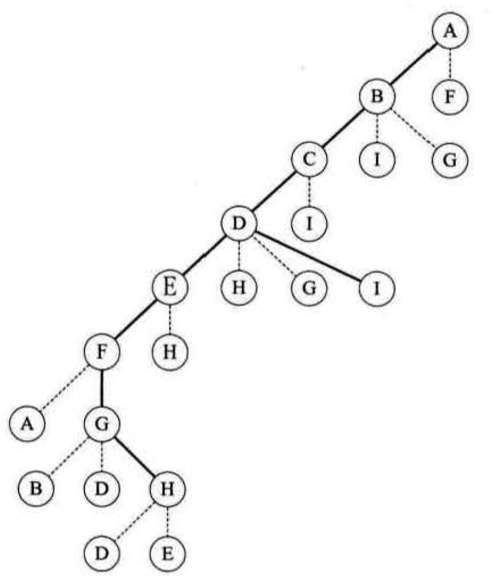实线是走过的路程，虚线就是我们的小人敲门然后发现标记过的一个过程，大家可以寄几模拟一哈。一句话总结就是：
从图中某个顶点 v 出发，访问此顶点，然后从 v 的未被访问的邻接点出发 深度优先遍历图结构，直至图中所有和 v 有路径相通的顶点都被访问到。
结构定义代码：
typedef char VertexType;
typedef int EdgeType;

#define MAXVEX 10
#define INFINITY 65535

typedef int boolean;
boolean visited[MAXVEX];

typedef struct
{
VertexType vexs[MAXVEX];
EdgeType arc[MAXVEX][MAXVEX];
int numVertexes,numEdges;
}MGraph;

邻接矩阵创建：
void CreateMGraph(MGraph *G)
{
int i,j,k;
printf("请输入顶点数和边数（空格隔开）\n");
scanf("%d %d",&G->numVertexes,&G->numEdges);
printf("请依次输入每个顶点的内容：\n");
for(i = 0;i < G->numVertexes;i++)
{
scanf("%c",&G->vexs[i]);
}
for(i = 0;i < G->numVertexes;i++)
{
for(j = 0;j < G->numVertexes;j++)
{
G->arc[i][j] = INFINITY;
}
}
for(k = 0;k < G->numEdges;k++)
{
printf("输入边（vi,vj）上的下标i，下标j：\n");
scanf("%d %d",&i,&j);
G->arc[i][j] = 1;
G->arc[j][i] = G->arc[i][j];
}
}

DFS算法
void DFS(MGraph G,int i) //深度优先递归算法
{
int j;
visited[i] = 1;
printf("%c",G.vexs[i]);
for(j = 0;j < G.numVertexes;j++)
{
if(G.arc[i][j] == 1 && !visited[j])
DFS(G,j);
}
}
void DFStraverse(MGraph G)  //深度遍历
{
int i;
for(i = 0;i < G.numVertexes;i++)
visited[i] = 0;
for(i = 0;i < G.numVertexes;i++)
{
if(!visited[i])
DFS(G,i);
}
}

这种方法比较好理解在于使用循环进入函数再递归，可以保证以邻接矩阵为储存单位的每一个格子都被遍历到，且做好标注，那么用邻接矩阵的DFS算法时间复杂度可以想见是 O（n²），嵌套两重循环
我们来看下一种实现方式，这次我们使用的是邻接单链表
结构定义：
typedef int boolean;
boolean visited[MAXVEX];

typedef char VertexType;
typedef int EdgeType;

#define MAXVEX 10
#define INFINITY 65535

typedef struct EdgeNode //边表结构点
{
int adjvex;
struct EdgeNode *next;
}EdgeNode;

typedef struct VertexNode //顶点表结构点
{
VertexType data;
EdgeNode *firstedge;
}VertexNode,AdjList[MAXVEX];

typedef struct //总表结构
{
AdjList adjList;
int numVertexes,numEdges;
}GraphAdjList;

比邻接矩阵复杂一点，但是其结构只有三种，总表、定点表和边表
创建：
void CreateALGraph(GraphAdjList *G)
{
int i,j,k;
EdgeNode *e;
printf("请输入顶点数和边数（空格隔开）\n");
scanf("%d %d",&G->numVertexes,&G->numEdges);
for(i = 0;i < G->numVertexes;i++)
{
scanf("%c",&G->adjList[i].data);
G->adjList[i].firstedge = NULL;
}
for(k = 0;k < G->numVertexes;k++)
{
printf("输入边（vi,vj）上的下标i，下标j：\n");
scanf("%d %d",&i,&j);
e = (EdgeNode*)malloc(sizeof(EdgeNode));
e->adjvex=i;
e->next = adjList[j].firstedge;
adjList[j].firstedge = e;

e = (EdgeNode*)malloc(sizeof(EdgeNode));
e->adjvex=j;
e->next = adjList[i].firstedge;
adjList[i].firstedge = e;
}
}

DFS算法实现：
void DFS(GraphAdjList GL,int i)
{
EdgeNode *p;
visited[i] = 1;
printf("%c",GL->adjList[i].data);
while(p)
{
if(!visited[p->adjvex])
DFS(GL,p->adjvex);
p = p->next;
}
}

void DFStraverse(GraphAdjList GL)
{
int i;
for(i = 0;i < GL->numVertexes;i++)
visited[i] = 0;
for(i = 0;i < GL->numVertexes;i++)
{
if(!visited[i])
DFS(GL,i);
}
}

利用邻接表的方式能够实现相同效果的遍历，同时这种方法的算法时间复杂度为 O（n+e）
显然对于点多边少的稀疏图来说，邻接表结构使得算法在时间效率上大大提高。


展开全文数据结构
• Given two binary trees, write a function to check if they are equal or not.Two binary trees are considered equal if they are structurally identical and the nodes ...题意很简单，就是DFS深度优先遍历。代
• 深度优先遍历DFS，广度优先遍历BFS。 讲述他们的原理，思想，与完美python代码。 深度优先遍历DFS 深度优先遍历： 寻找初始点，并将该点压入一个栈Q中。（这里采用python的内置容器的list） pop弹出Q栈的末尾元素，...
• 紧接着上一个博客，补上了广度优先遍历 //数据结构之图的存储结构，初始化，遍历，应用 #include<iostream> #include <stdlib.h> #include<string> #include<stdio.h> using namespace ...图的数据结构
• 文章目录二叉树的深度优先遍历二叉树DFS的应用二叉树DFS（递归方式）[Java]二叉树DFS（非递归堆栈方式）[Java]返回二叉树所有路径 [Java]判断是否存在 路径长度为 target 的路径 [Java]返回路径 和 为 target 的所有...算法 数据结构 java
• 广度优先搜索(Breadth First Search，简称BFS) 从某个节点出发，首先访问该节点，然后依次从它...深度优先搜索(Depth First Search，简称DFS) 从某节点出发，在访问了这个节点之后依次访问这个节点的各个未曾访问...广度优先遍历
• 文章目录一、DFS1.DFS原理2.剪枝二、树的DFS1.部分和问题三、矩阵迷宫1.八联通 一、DFS 1.DFS原理 DFS（Depth First Search） 从某个状态开始，不断地转移状态直到无法转移，然后退回到前一步的状态，继续转移到...
• DFS的大概模板 void dfs(int x)//关于传入参数问题，根据题意而定，看在题目运行的过程中，哪些是在变得 { if(满足输出条件) { 输出解; return ; } if(目前已经没有必要进行下去的条件){ return ; }//剪枝...
• vis[vec[root][i]]) { int tmp=dfs(vec[root][i],level+1); if(tmp>max) max=tmp; } } return max; } int main() { cin>>n; if(n==1) { cout; return 0; } for(int i=0;i;i++) { int x,y; ...PAT
• 今天看到了数据结构的图中的深度优先遍历，一共有两种形式，邻接矩阵和邻接表，下面给出两种方式的代码 具体算法原理书上讲的很清楚，在此不赘述了。（小白的dfs笔记） 邻接矩阵的代码： #include <iostream> ...数据结构
• 下面是C++的做法，就是一个简单的BFS广度优先遍历的做法 代码如下： #include #include #include #include #include #include #include #include #include #include #include...leetcode...